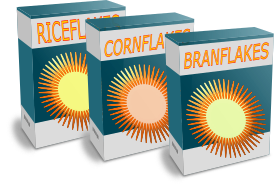# Exam-Style Questions on Fractions

## Problems on Fractions adapted from questions set in previous Mathematics exams.

### 1.

IGCSE Core

Without using your calculator, work out the value of the following calculations giving each answer as a fraction in its lowest terms. You must show all your working.

(a)

$$1\frac{5}{12} - \frac58$$

(b)

$$\frac49 ÷ 6\frac23$$

### 2.

IGCSE Extended

In January, a supermarket sold 12 624 boxes of breakfast cereal.

(a) The cost of a box of cereal was £2.15 . Calculate the amount received from the sale of the 12 624 boxes. Give your answer correct to the nearest hundred pounds.

(b) The number of boxes sold in February was 13% more than the number sold in January. Calculate the number of boxes sold in February.(c) There were 3 different types of cereal. The number of boxes sold of each type every month was in the ratio Riceflakes : Cornflakes : Branflakes = 4 : 5 : 3. The total number of boxes sold was 12 624. Calculate the number of boxes of Branflakes sold.

(d) How may more boxes of Cornflakes than Riceflakes were sold in February?

(e) $$\frac{5}{6}$$ of the 12 624 bottles sold in January are included in a special promotion. Calculate the number of boxes that are included in that promotion.

### 3.

GCSE Higher

Find the product of the two numbers below giving your answer as a mixed number in its simplest form.

 $$3 \frac{2}{3}$$ $$4 \frac{2}{5}$$

### 4.

GCSE Higher

Unit fractions have numerators equal to 1, for example $$\frac12$$,$$\frac19$$ and $$\frac{1}{36}$$.

Unit fractions can be written as the sum of two different unit fractions, for example

$$\frac12 = \frac13 + \frac16$$

Write each of the following unit fractions as the sum of two different unit fractions.

(a) $$\frac14$$

(b) $$\frac16$$

(c) $$\frac19$$

If you would like space on the right of the question to write out the solution try this Thinning Feature. It will collapse the text into the left half of your screen but large diagrams will remain unchanged.

The exam-style questions appearing on this site are based on those set in previous examinations (or sample assessment papers for future examinations) by the major examination boards. The wording, diagrams and figures used in these questions have been changed from the originals so that students can have fresh, relevant problem solving practice even if they have previously worked through the related exam paper.

The solutions to the questions on this website are only available to those who have a Transum Subscription.

Exam-Style Questions Main Page

Search for exam-style questions containing a particular word or phrase:

To search the entire Transum website use the search box in the grey area below.